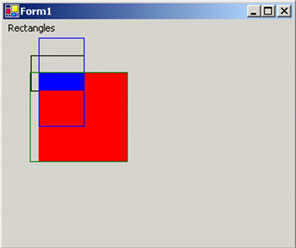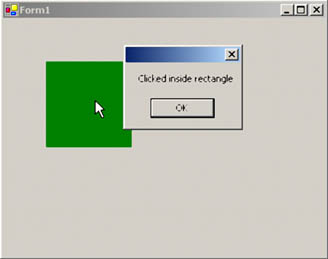# The Rectangle Structure

In Chapter 2 we discussed the Rectangle and RectangleF structures, and how to use their properties and methods. In this chapter we will discuss the functionality that we missed in Chapter 2. To refresh your memory, let's take a quick look at the Rectangle structure.

A Rectangle object stores the top left corner and height and width of a rectangular region. In this section we will see how to create and use the Rectangle structure.

6.1.1 Constructing a Rectangle Object

There are several ways to create a Rectangle object. For example, you can create a Rectangle object from four integer values representing the starting point and size of the rectangle, or from Point and Size structures. Listing 6.1 creates Rectangle objects from Size, Point, and direct values. As this code shows, a Rectangle constructor can take a Point and a Size object or, alternatively, the starting point (as separate variables x and y), width, and height.

Listing 6.1 Constructing Rectangle objects

```int x = 20;
int y = 30;
int height = 30;
int width = 30;
// Create a starting point
Point pt = new Point(10, 10);
// Create a size
Size sz = new Size(60, 40);
// Create a rectangle from a point
// and a size
Rectangle rect1 = new Rectangle(pt, sz);
Rectangle rect2 =
new Rectangle(x, y, width, height);
```

6.1.2 Constructing a RectangleF Object

You can also create a RectangleF object in several ways: from four floating point numbers with the starting and ending points and height and width of the rectangle, or from a point and a size. RectangleF is a mirror of Rectangle, including properties and methods. The only difference is that RectangleF takes floating point values. For example, instead of Size and Point, RectangleF uses SizeF and PointF. Listing 6.2 creates RectangleF objects in two different ways.

Listing 6.2 Constructing RectangleF objects

```// Create a starting point
PointF pt = new PointF(30.8f, 20.7f);
// Create a size
SizeF sz = new SizeF(60.0f, 40.0f);
// Create a rectangle from a point and
// a size
RectangleF rect1 = new RectangleF(pt, sz);
// Create a rectangle from floating points
RectangleF rect2 =
new RectangleF(40.2f, 40.6f, 100.5f, 100.0f);
```

6.1.3 Rectangle Properties and Methods

The Rectangle structure provides properties that include Bottom, Top, Left, Right, Height, Width, IsEmpty, Location, Size, X, and Y. Listing 6.3 creates two rectangles (rect1 and rect2), reads these properties, and displays their values in a message box.

Listing 6.3 Using the the Rectangle structure properties

```private void PropertiesMenu_Click(object sender,
System.EventArgs e)
{
// Create a point
PointF pt = new PointF(30.8f, 20.7f);
// Create a size
SizeF sz = new SizeF(60.0f, 40.0f);
// Create a rectangle from a point and
// a size
RectangleF rect1 = new RectangleF(pt, sz);
// Create a rectangle from floating points
RectangleF rect2 =
new RectangleF(40.2f, 40.6f, 100.5f, 100.0f);
// If rectangle is empty,
// set its Location, Width, and Height
// properties
if (rect1.IsEmpty)
{
rect1.Location = pt;
rect1.Width = sz.Width;
rect1.Height = sz.Height;
}
string str =
"Location:"+ rect1.Location.ToString();
str += "X:"+rect1.X.ToString() + "
";
str += "Y:"+ rect1.Y.ToString() + "
";
str += "Left:"+ rect1.Left.ToString() + "
";
str += "Right:"+ rect1.Right.ToString() + "
";
str += "Top:"+ rect1.Top.ToString() + "
";
str += "Bottom:"+ rect1.Bottom.ToString();
MessageBox.Show(str);
}
```

As we discussed in Chapter 2, the Rectangle structure provides methods that include Round, Truncate, Inflate, Ceiling, Intersect, and Union.

• The Round method converts a RectangleF object to a Rectangle object by rounding off the values of RectangleF to the nearest integer.
• The Truncate method converts a RectangleF object to a Rectangle object by truncating the values of RectangleF.
• The Inflate method creates a rectangle inflated by the specified amount.
• The Ceiling method converts a RectangleF object to a Rectangle object by rounding to the next higher integer values.
• The Intersect method replaces a rectangle by its intersection with a supplied rectangle.
• The Union method gets a rectangle that contains the union of two rectangles.

Listing 6.4 shows how to use the Round, Truncate, Inflate, Ceiling, Intersect, and Union methods.

Listing 6.4 Using the Rectangle structure methods

```private void MethodsMenu_Click(object sender,
System.EventArgs e)
{
// Create a Graphics object
Graphics g = this.CreateGraphics();
// Create a point and a size
PointF pt = new PointF(30.8f, 20.7f);
SizeF sz = new SizeF(60.0f, 40.0f);
// Create two rectangles
RectangleF rect1 = new RectangleF(pt, sz);
RectangleF rect2 =
new RectangleF(40.2f, 40.6f, 100.5f, 100.0f);
// Ceiling a rectangle
Rectangle rect3 = Rectangle.Ceiling(rect1);
// Truncate a rectangle
Rectangle rect4 = Rectangle.Truncate(rect1);
// Round a rectangle
Rectangle rect5 = Rectangle.Round(rect2);
// Draw rectangles
g.DrawRectangle(Pens.Black, rect3);
g.FillRectangle(Brushes.Red, rect5);
// Intersect a rectangle
Rectangle isectRect =
Rectangle.Intersect(rect3, rect5);
// Fill rectangle
g.FillRectangle(
new SolidBrush(Color.Blue), isectRect);
// Inflate a rectangle
Size inflateSize = new Size(0, 40);
isectRect.Inflate(inflateSize);
// Draw rectangle
g.DrawRectangle(Pens.Blue, isectRect);
// Empty rectangle and set its properties
rect4 = Rectangle.Empty;
rect4.Location = new Point(50, 50);
rect4.X = 30;
rect4.Y = 40;
// Union rectangles
Rectangle unionRect =
Rectangle.Union(rect4, rect5);
// Draw rectangle
g.DrawRectangle(Pens.Green, unionRect);
// Displose of objects
g.Dispose();
}
```

Figure 6.3 shows the output of Listing 6.3.

Figure 6.3. Using Rectangle methods6.1.3.1 The Contains Method and Hit Test

The Contains method is used to determine whether a rectangle or point is inside the current rectangle. If a point is inside the current rectangle, the Contains method returns true; otherwise it returns false. One of the common uses of Contains is to find out if a mouse button was clicked inside a rectangle.

6.1.3.2 Hit Test Example

To see proper use of the Contains method, let's create a Windows application and draw a rectangle on the form. Whether the user clicks inside or outside of the rectangle, we will have the application generate an appropriate message.

First we define a class-level Rectangle variable as follows:

```
```
```Rectangle bigRect = new Rectangle(50, 50, 100, 100);
```
```
```

Then we use the form's paint event handler because we want to render graphics whenever the form needs to refresh. The form's paint event handler code looks like this:

```
```
```private void Form1_Paint(object sender,
System.Windows.Forms.PaintEventArgs e)
{
SolidBrush brush = new SolidBrush(Color.Green);
e.Graphics.FillRectangle(brush, bigRect);
brush.Dispose();
}
```
```
```

Our last step is to determine whether the user clicked inside the rectangle. We track the user's mouse-down event and write code for the left mouse button click event handler. The MouseEventArgs enumeration provides members to find out which mouse button is clicked. The MouseButtons enumeration has members that include Left, Middle, None, Right, Xbutton1, and Xbutton2, which represent the mouse buttons.

We check to see if the mouse button clicked was the left button, then create a rectangle, and (if the mouse button was clicked) generate a message. Listing 6.5 shows the code for this process.

Listing 6.5 Determining whether a mouse was clicked inside a rectangle

```private void Form1_MouseDown(object sender,
System.Windows.Forms.MouseEventArgs e)
{
if(e.Button == MouseButtons.Left)
{
if (bigRect.Contains( new Point(e.X, e.Y)) )
MessageBox.Show("Clicked inside rectangle");
else
MessageBox.Show("Clicked outside rectangle");
}
}
```

When you run the application and click on the rectangle, the output looks like Figure 6.4.

Figure 6.4. Hit test using the Contains methodThe Contains method also allows us to find out whether a rectangle fits inside another rectangle. Listing 6.6 checks whether smallRect is within bigRect.

Listing 6.6 Checking if one rectangle is within another

```Point pt = new Point(0, 0);
Size sz = new Size(200, 200);
Rectangle bigRect = new Rectangle(pt, sz);
Rectangle smallRect = new Rectangle(30, 20, 100, 100);
if (bigRect.Contains(smallRect) )
MessageBox.Show("Rectangle "+smallRect.ToString()
+" is inside Rectangle "+ bigRect.ToString() );
```GDI+ Programming with C#
ISBN: 073561265X
EAN: N/A
Year: 2003
Pages: 145